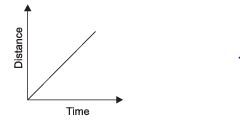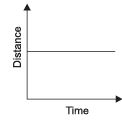Courses

# Very Short Answers - Motion, Science, Class 9 Class 9 Notes | EduRev

## Class 9 : Very Short Answers - Motion, Science, Class 9 Class 9 Notes | EduRev

The document Very Short Answers - Motion, Science, Class 9 Class 9 Notes | EduRev is a part of the Class 9 Course Class 9 Science by VP Classes.
All you need of Class 9 at this link: Class 9

Q1. Define uniform motion.
Ans.
When an object covers equal distances in equal intervals of time, it is said to be in uniform motion.

Q2. Define speed.
Ans.
It is defined as the distance travelled by an object in unit time. Its unit is m/s.

Q3. Define average speed.
Ans.
The total distance travelled by an object divided by the total time taken.

Q4. Define velocity.
Ans.
Velocity is the speed of an object moving in definite direction.

Q5. Define acceleration.
Ans.
Change in the velocity of an object per unit time.

a= (v-u)/t, S.I unit in m/s2

Q6. While plotting a distance-time graph, why do we plot time on x-axis.
Ans.
While plotting a distance-time graph, we should plot time on x-axis as, all independent quantities are plotted on x-axis.

Q7. What is odometer?
Ans.
Odometer is a device fitted in the automobiles to show the distance travelled.

Q8. Draw a distance-time graph that represents uniform speed.
Ans.Q9. What does the area under velocity-time graph represent?
Ans.
The area under the velocity-time graph represents the displacement.

Q10. A body travels a distance from A to B its physical quantity is measured to be −15 m/s. Is it speed or velocity? Give reason for the answer.
Ans.
The physical quantity is – 15 m/s. It represents velocity because sign of speed cannot be a negative. Negative sign indicates the opposite direction.

Q11. With the help of distance-time graph show that the object is stationary.
Ans.Q12. What type of velocity-time graph will you get for a particle moving with a constant acceleration?
Ans.
For a particle moving with a constant acceleration velocity-time graph will be a straight line inclined to x-axis.

Q13. Define uniform circular motion.
Ans
. When a body moves in a circular path with uniform speed, its motion is called uniform circular motion.

Q14. What is deceleration?
Ans.
When the speed of an object decreases, the object is said to be decelerating. In this case the direction of acceleration is opposite to the velocity of the object.

Q15. A car travels with a speed of 30 km/h and then returns back with a speed of 50 km/h. Find average speed of the car.
Ans.
Average speed = ((30+50)/2)= 40 km/h.

Offer running on EduRev: Apply code STAYHOME200 to get INR 200 off on our premium plan EduRev Infinity!

75 docs|14 tests

,

,

,

,

,

,

,

,

,

,

,

,

,

,

,

,

,

,

,

,

,

,

,

,

,

,

,

;